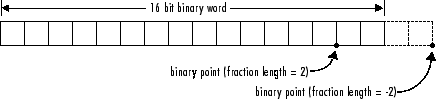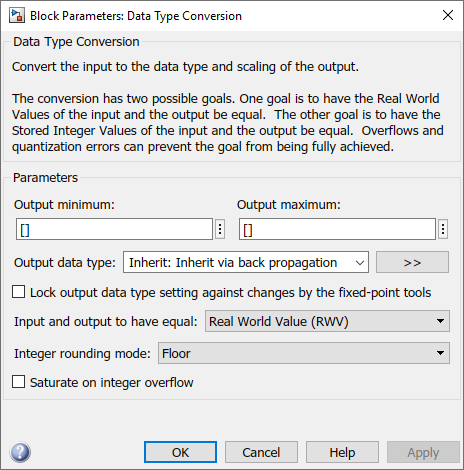Main Content

## Configure Blocks with Fixed-Point Output

To create a fixed-point model, configure Simulink® blocks to output fixed-point signals. Simulink blocks that support fixed-point output provide parameters that allow you to specify whether a block should output fixed-point signals and, if so, the size, scaling, and other attributes of the fixed-point output. These parameters typically appear on the Signal Attributes pane of the block's parameter dialog box.The following sections explain how to use these parameters to configure a block for fixed-point output.

### Specify the Output Data Type and Scaling

Many Simulink blocks allow you to specify an output data type and scaling using a parameter that appears on the block dialog box. This parameter (typically named Output data type) provides a pull-down menu that lists the data types a particular block supports. In general, you can specify the output data type as a rule that inherits a data type, a built-in data type, an expression that evaluates to a data type, or a Simulink data type object. For more information, see Control Signal Data Types.

The Fixed-Point Designer™ software enables you to configure Simulink blocks with:

• Fixed-point data types

Fixed-point data types are characterized by their word size in bits and by their binary point — the means by which fixed-point values are scaled.

• Floating-point data types

Floating-point data types are characterized by their sign bit, fraction (mantissa) field, and exponent field.

To configure blocks with Fixed-Point Designer data types, specify the data type parameter on a block dialog box as an expression that evaluates to a data type. Alternatively, you can use an assistant that simplifies the task of entering data type expressions (see Specify Fixed-Point Data Types with the Data Type Assistant). The sections that follow describe varieties of fixed-point and floating-point data types, and the corresponding functions that you use to specify them.

#### Integers

To specify unsigned and signed integers, use the `uint` and `sint` functions, respectively.

For example, to configure a 16-bit unsigned integer via the block dialog box, specify the Output data type parameter as `uint(16)`. To configure a 16-bit signed integer, specify the Output data type parameter as `sint(16)`.

For integer data types, the default binary point is assumed to lie to the right of all bits.

#### Fractional Numbers

To specify unsigned and signed fractional numbers, use the `ufrac` and `sfrac` functions, respectively.

For example, to configure the output as a 16-bit unsigned fractional number via the block dialog box, specify the Output data type parameter to be `ufrac(16)`. To configure a 16-bit signed fractional number, specify Output data type to be `sfrac(16)`.

Fractional numbers are distinguished from integers by their default scaling. Whereas signed and unsigned integer data types have a default binary point to the right of all bits, unsigned fractional data types have a default binary point to the left of all bits, while signed fractional data types have a default binary point to the right of the sign bit.

Both unsigned and signed fractional data types support guard bits, which act to guard against overflow. For example, `sfrac(16,4)` specifies a 16-bit signed fractional number with 4 guard bits. The guard bits lie to the left of the default binary point.

#### Generalized Fixed-Point Numbers

You can specify unsigned and signed generalized fixed-point numbers with the `ufix` and `sfix` functions, respectively.

For example, to configure the output as a 16-bit unsigned generalized fixed-point number via the block dialog box, specify the Output data type parameter to be `ufix(16)`. To configure a 16-bit signed generalized fixed-point number, specify Output data type to be `sfix(16)`.

Generalized fixed-point numbers are distinguished from integers and fractionals by the absence of a default scaling. For these data types, a block typically inherits its scaling from another block.

Note

Alternatively, you can use the `fixdt` function to create integer, fractional, and generalized fixed-point objects. The `fixdt` function also allows you to specify scaling for fixed-point data types.

#### Floating-Point Numbers

The Fixed-Point Designer software supports single-precision and double-precision floating-point numbers as defined by the IEEE® Standard 754–1985 for Binary Floating-Point Arithmetic. You can specify floating-point numbers with the Simulink `float` function.

For example, to configure the output as a single-precision floating-point number via the block dialog box, specify the Output data type parameter as `float('single')`. To configure a double-precision floating-point number, specify Output data type as `float('double')`.

### Specify Fixed-Point Data Types with the Data Type Assistant

The Data Type Assistant is an interactive graphical tool that simplifies the task of specifying data types for Simulink blocks and data objects. The assistant appears on block and object dialog boxes, adjacent to parameters that provide data type control, such as the Output data type parameter. For more information about accessing and interacting with the assistant, see Specify Data Types Using Data Type Assistant.

You can use the Data Type Assistant to specify a fixed-point data type. When you select `Fixed point` in the Mode field, the assistant displays fields for describing additional attributes of a fixed-point data type, as shown in this example:You can set the following fixed-point attributes:

#### Signedness

Select whether you want the fixed-point data to be `Signed` or `Unsigned`. Signed data can represent positive and negative quantities. Unsigned data represents positive values only.

#### Word Length

Specify the size (in bits) of the word that will hold the quantized integer. Large word sizes represent large quantities with greater precision than small word sizes. Fixed-point word sizes up to 128 bits are supported for simulation.

#### Scaling

Specify the method for scaling your fixed-point data to avoid overflow conditions and minimize quantization errors. You can select the following scaling modes:

Scaling ModeDescription
`Binary point`

If you select this mode, the assistant displays the Fraction length field, specifying the binary point location.

Binary points can be positive or negative integers. A positive integer moves the binary point left of the rightmost bit by that amount. For example, an entry of 2 sets the binary point in front of the second bit from the right. A negative integer moves the binary point further right of the rightmost bit by that amount.See Binary-Point-Only Scaling for more information.

`Slope and bias`

If you select this mode, the assistant displays fields for entering the Slope and Bias.

• Slope can be any positive real number.

• Bias can be any real number.

See Slope and Bias Scaling for more information.

`Best precision`

If you select this mode, the block scales a constant vector or matrix such that the precision of its elements is maximized. This mode is available only for particular blocks.

See Constant Scaling for Best Precision for more information.

#### Calculate Best-Precision Scaling

The Fixed-Point Designer software can automatically calculate “best-precision” values for both ```Binary point``` and `Slope and bias` scaling, based on the values that you specify for other parameters on the dialog box. To calculate best-precision-scaling values automatically, enter values for the block's Output minimum and Output maximum parameters. Then click the button in the assistant.

### Rounding

You specify how fixed-point numbers are rounded with the Integer rounding mode parameter. The following rounding modes are supported:

• `Ceiling` — This mode rounds toward positive infinity and is equivalent to the MATLAB® `ceil` function.

• `Convergent` — This mode rounds toward the nearest representable number, with ties rounding to the nearest even integer. Convergent rounding is equivalent to the Fixed-Point Designer `convergent` function.

• `Floor` — This mode rounds toward negative infinity and is equivalent to the MATLAB `floor` function.

• `Nearest` — This mode rounds toward the nearest representable number, with the exact midpoint rounded toward positive infinity. Rounding toward nearest is equivalent to the Fixed-Point Designer `nearest` function.

• `Round` — This mode rounds to the nearest representable number, with ties for positive numbers rounding in the direction of positive infinity and ties for negative numbers rounding in the direction of negative infinity. This mode is equivalent to the Fixed-Point Designer `round` function.

• `Simplest` — This mode automatically chooses between round toward floor and round toward zero to produce generated code that is as efficient as possible.

• `Zero` — This mode rounds toward zero and is equivalent to the MATLAB `fix` function.

For more information about each of these rounding modes, see Rounding.

### Overflow Handling

To control how overflow conditions are handled for fixed-point operations, use the Saturate on integer overflow check box.

If this box is selected, overflows saturate to either the maximum or minimum value represented by the data type. For example, an overflow associated with a signed 8-bit integer can saturate to -128 or 127.

If this box is not selected, overflows wrap to the appropriate value that is representable by the data type. For example, the number 130 does not fit in a signed 8-bit integer, and would wrap to -126.

### Lock the Output Data Type Setting

If the output data type is a generalized fixed-point number, you have the option of locking its output data type setting by selecting the Lock output data type setting against changes by the fixed-point tools check box.

When locked, the Fixed-Point Tool and automatic scaling script `autofixexp` do not change the output data type setting. Otherwise, the Fixed-Point Tool and `autofixexp` script are free to adjust the output data type setting.

### Real-World Values Versus Stored Integer Values

You can configure Data Type Conversion blocks to treat signals as real-world values or as stored integers with the Input and output to have equal parameter.The possible values are `Real World Value (RWV)` and `Stored Integer (SI)`.

In terms of the variables defined in Scaling, the real-world value is given by V and the stored integer value is given by Q. You may want to treat numbers as stored integer values if you are modeling hardware that produces integers as output.

## SupportGet trial now